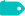# 差分放大器计算公式和工作原理

15573 2022-04-15 来源: IC先生 作者: IC先生

### 差分放大器的概念### 运算放大器输出电压计算公式

V OUT+ = V + G+

G+ = (R3 + R4 ) / R3 = 1 + (R4 / R3 )

V OUT+ = V 1 (R2 / R1 + R2 ) (1 + (R4 / R3 ))

G – = –R4/R3

V OUT– = V2 (– R4/R3 )

V OUT = V OUT + + V OUT–

V OUT = V1 (R2/R 1 + R2 ) (1 + (R4/R3 )) – V2 (R4/R3 )

V OUT = R2/R1 (V1 – V2 ) = R4/R3 (V1 – V2 )

### 差分放大器传递函数计算公式

Vout=v1.R2/R1+R2(1+R4/R3)-V2.R4/R3Vout2=-V2.(R4/R3)Vout1=V.(1+R4/R3)

R1、R2 电阻器是V1的衰减器，因此V可以按以下等式确定：

V=V1.R2/R1+R2

Vout1=V1.R2/R1+R2.(1+R4/R3)### 差分放大器的重要参数

• 增益
• 共模输入
• 共模抑制比 (CMRR)
1、增益

V OUT = R 2 / R 1 (V 1 – V 2 )

A D = V OUT / (V 1 – V 2 ) = R 2 / R 1

2、共模输入

V DM = V 1 – V 2

V CM = (V 1 + V 2 ) / 2

V 1 = V CM + V DM / 2和V 2 = V CM – V DM / 2V OUT = A D V DM + A C V CM，其中 V DM是电压差V 1 – V 2，V CM是共模电压 (V 1 + V 2 ) / 2，而A D和A C分别是差模和共模增益。

3、共模抑制比 (CMRR)

CMRR = A D / A C• 高差分电压增益
• 低共模增益
• 高输入阻抗
• 低输出阻抗
• 高CMRR
• 高带宽
• 低失调电压和电流

### 示例：使用惠斯通电桥的差分放大器### 总结